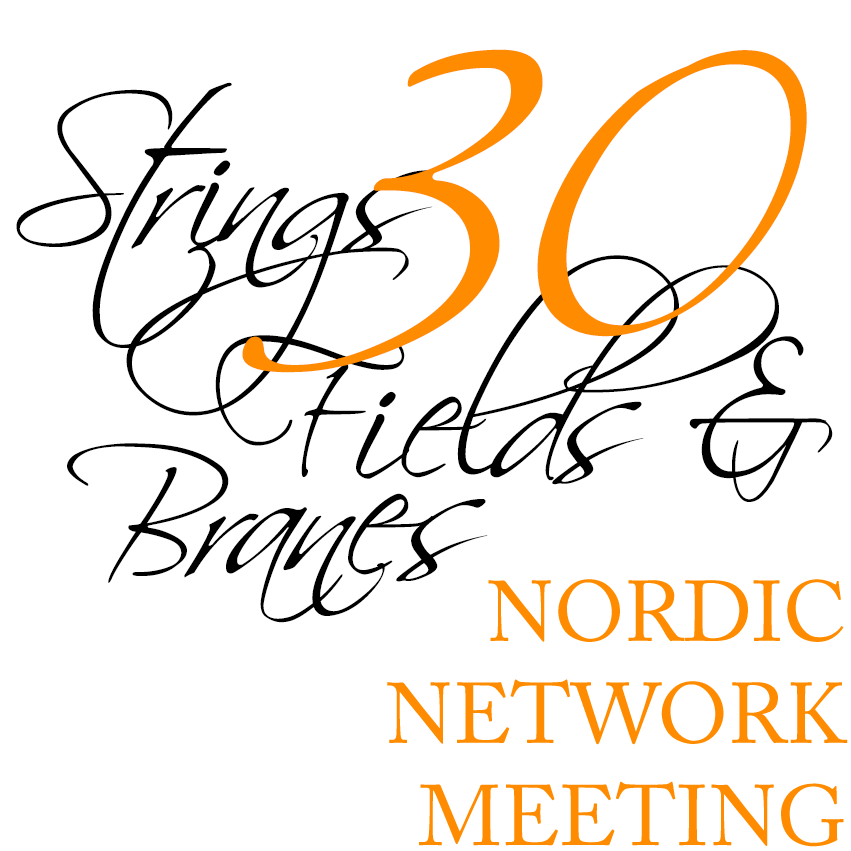#30th Nordic Network Meeting on "Strings, Fields, and Branes"

Nov 22 – 24, 2021
Nordita, Stockholm
Europe/Stockholm timezone

## Quarter-BPS operators in $\mathcal{N}=4$ SYM: a case study

Nov 22, 2021, 4:40 PM
20m
room 4204 (Nordita, Stockholm)

Short talk

### Speaker

Giulia Fardelli (Uppsala University)

### Description

In this work we start a systematic study of quarter-BPS operators in $\mathcal{N}=4$ SYM with gauge group SU($N$) at large $N$. In particular we consider $\mathcal{O}_{pq}$, operators transforming in the $[q,p,q]$ representation of the SU(4) R-symmetry: when expanded in $\mathcal{N}=2$ supermultiplets, they contain Schur operators making it possible to derive the Ward Identities through the chiral algebra. Our focus will be on the simplest non trivial example, namely the operator in the $[2,0,2]$ representation. In the planar limit this is a double trace operator and, because of that, its OPE analysis will give access to unexplored information on higher trace operators, whose knowledge is crucial to investigate $\mathcal{N}=4$ SYM away from the leading orders in the large $N$ expansion.
I will discuss the results that we obtained in this context by means of modern analytic CFT techniques such as chiral algebra and Lorentzian inversion formula.

### Primary author

Giulia Fardelli (Uppsala University)

### Co-authors

Agnese Bissi (Uppsala University) Dr Andrea Manenti (Uppsala University)